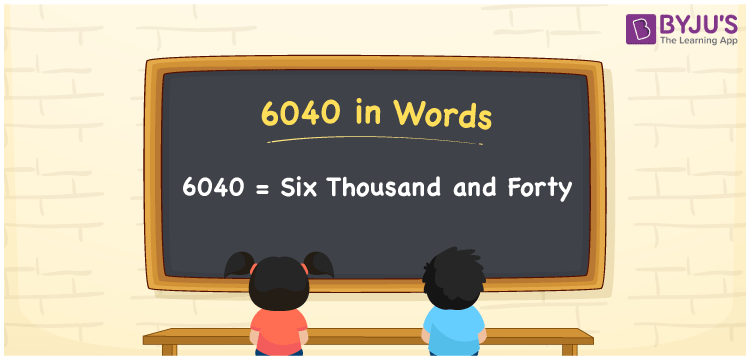# 6040 in Words

We can write 6040 in words as Six thousand and forty. If you bought a silver coin worth Rs. 6040, then you can say, “I bought a silver coin for Six thousand and forty rupees”. Thus, we employ the word form of cardinal numbers to communicate a specific quantity or numerical amount.

 6040 in words Six thousand and forty Six thousand and forty in Numbers 6040

## 6040 in English Words

We generally write numbers in words using the English alphabet. So, we spell 6040 in English as “Six thousand and forty”.## How to Write 6040 in Words?

The number 6040 has four digits, so we need to create a place value chart with four columns, as given below. From this, we can easily derive the word form of 6040.

 Thousands Hundreds Tens Ones 6 0 4 0

Here, ones = 0, tens = 4, hundreds = 0, and thousands = 6.

These numbers can be expanded as follows.

6 × Thousand + 0 × Hundred + 4 × Ten + 0 × One

= 6 × 1000 + 0 × 100 + 4 × 10 + 0 × 1

= 6000 + 40

= Six thousand + Forty

= Six thousand and forty

Therefore, 6040 in words = Six thousand and forty.

6040 is a natural number that precedes 6041 and succeeds 6039.

6040 in words – Six thousand and forty

Is 6040 an odd number? – No

Is 6040 an even number? – Yes

Is 6040 a perfect square number? – No

Is 6040 a perfect cube number? – No

Is 6040 a prime number? – No

Is 6040 a composite number? – Yes

## Frequently Asked Questions on 6040 in Words

Q1

### How do you say 6040 in words?

You can spell the number 6040 in English words as Six thousand and forty.
Q2

### Write Rs. 6040 in words.

We can write Rs. 6040 in words as “Six thousand and forty rupees”.
Q3

### What is the number name of 6040?

The number name of 6040 is Six thousand and forty. Therefore, 6040 = Six thousand and forty.